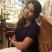# DILR Preparation Tips for MBA Number Systems: Definition, Rules, Sample Questions, Tips and FAQs

DILR Preparation Tips for MBA 2022

9.1K Views
This exam is conducted for courses given below:Vipra ShrivastavaSenior Manager - Content

Updated on May 9, 2022 16:06 IST

## What is Number Systems?

The Number System is the method by which we formulate numbers. There are several forms of number systems including binary, decimal, etc. It is a system wherein numbers are expressed. It is the mathematical notation for describing numbers with a consistent sequence of digits or symbols. It proceeds to represent any number and the arrangement of the figures.

Number System Concepts

Under Number Systems, we learn about:

• Natural Numbers

• Whole Numbers

• Integers

• Rational Numbers

• Irrational Numbers

• Real Numbers

Questions on Number Systems with Solutions

1. What are the five numbers between one and two?

There are 5 rational numbers between one and two.

Solution: We need to find 5 rational numbers between 1 and 2

Divide and multiple both the numbers by (5+1)

Hence,

6/6 and 12/6 are rational numbers now.

Therefore, 6/6, 7/6, 8/6, 9/6, 10/6, 11/6, 12/6.

Irrational numbers cannot be represented as either a fraction or a decimal.

2. Is there a number √3 on the number line?

Solution:

To locate √3 on the number line, we need to:

Build BD that passes via point OB perpendicular to it.

Using Pythagora's theorem, we notice that OD = √((√2)^2+1^2) = √3.

Draw an arc with centre O and radius OD to cut the number line at Q.

## Terminating and Non-Terminating Decimal Expansion

A rational number either has a finite expansion into rational numbers, or it continues forever.

The decimal expansion of an irrational number is not terminating or recurring.

1. Demonstrate that 0.3333 … = 0.3, may be represented in rational form; i.e, p/q.

Let x = 0.33333.

10 x = 10 × (0.333….) = 3.333....

3.3333... = 3 + x.

10x = 3 + x.

9/x = 3.

x = ⅓.

2. Write the following in decimal form and name expansions.

1. 36/100.

2. 1/11.

Solution

1. 36/100 = 0.36.

It is terminating.

1. 1/11 = 0.09090909…

The solution is non-terminating and repeating.

Illustrated Examples

1. Multiply 5√3 by 4√3.

Solution: 5√3 x 4√3

5 x 4 x √3 x √3

= 20 x 3

= 60

2. Rationalise the denominator of √3/(√2-√7).

Solution: Multiply both numerator and denominator by √2+√7

[√3/(√2-√7)] x [(√2+√7)/(√2+√7)]

Numerator = √3(√2+√7)

Denominator = (√2-√7)(√2+√7) = (√2)^2-(√7)^2 = 2-7 = -5

Therefore,

[√3(√2+√7)]/-5

= -√3/5(√2+√7)

3. Rationalise the denominator of 1/√5.

Solution: To rationalise the denominator of 1/√5, we need to multiply the numerator and denominator by √5

1/√5 x (√5/√5)

= √5/5

## FAQs Regarding Number Systems

Q: What is the significance of number systems?

A: In mathematics, a number system is characterised as a framework that allows all numbers to be represented. The number method offers a distinct means of communicating numbers and the logical basis for a math equation.

Q: Describe the various groups of numbers.

A: The various kinds of numbers are:
• Natural numbers
• Whole numbers
• Real numbers
• Rational numbers
• Irrational numbers
• Complex numbers

Q: Why are the numbers used?

A: The numbers define the spectrum of everything. Numbers are used to measuring anything from income to time. Without statistics, we cannot realise how useful items are.

Q: What are the four different types of number systems?

A: The four major types of number system are:
• Binary number system (base 2 number system)
• Octal number system (base 8 number system)
• Decimal number system (base 10 number system)
• Hexadecimal number system (base 16 number system)

Q: What are the uses of number systems?

A: The most popular usage of the number system is in computers. The binary number system is used. Binary numbers are used in the encoding process of digital.

## Explore Other Exams

 23 Sep '22 - 26 Sep '22 CAT 2022 form correction proce... 27 Oct '22 CAT 2022 admit card download
 19 May '22 CUET 2022 Session IV Results 15 May '22 PI and Micro Presentation (Ses...
 11 Sep '22 MAH MBA CET result 2022 23 Aug '22 - 25 Aug '22 MAH CET 2022 exam
 18 Sep '22 MAT 2022 CBT 13 Sep '22 MAT 2022 Admit Card CBT Downlo...
 19 May '18 TANCET MBA 2018 exam 23 Apr '18 TANCET MBA 2018 registrations ...
 17 Aug '22 Result declaration 12 Aug '22 Last day of submission of Cros...
The list of exams are recommended based on what other exams do the students in your area show interest in